- BetterExplained - https://betterexplained.com -

# Developing Your Intuition For Math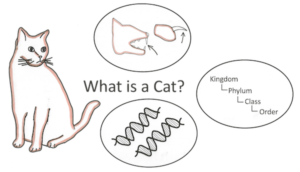Our initial exposure to an idea shapes our intuition. And our intuition impacts how much we enjoy a subject. What do I mean?

Suppose we want to define a “cat”:

• Caveman definition: A furry animal with claws, teeth, a tail, 4 legs, that purrs when happy and hisses when angry…
• Evolutionary definition: Mammalian descendants of a certain species (F. catus), sharing certain characteristics…
• Modern definition: You call those definitions? Cats are animals sharing the following DNA: ACATACATACATACAT…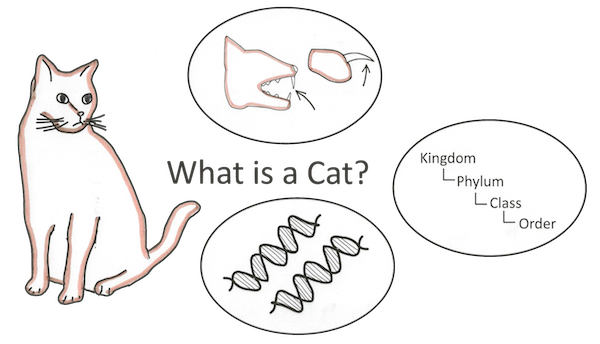(Illustration courtesy Common Craft)

The modern definition is precise, sure. But is it the best? Is it what you’d teach a child learning the word? Does it give better insight into the “catness” of the animal? Not really. The modern definition is useful, but after getting an understanding of what a cat is. It shouldn’t be our starting point.

Unfortunately, math understanding seems to follow the DNA pattern. We’re taught the modern, rigorous definition and not the insights that led up to it. We’re left with arcane formulas (DNA) but little understanding of what the idea is.

Let’s approach ideas from a different angle. I imagine a circle: the center is the idea you’re studying, and along the outside are the facts describing it. We start in one corner, with one fact or insight, and work our way around to develop our understanding. Cats have common physical traits leads to Cats have a common ancestor leads to A species can be identified by certain portions of DNA. Aha! I can see how the modern definition evolved from the caveman one.

But not all starting points are equal. The right perspective makes math click — and the mathematical “cavemen” who first found an idea often had an enlightening viewpoint. Let’s learn how to build our intuition.

## What is a Circle?

Time for a math example: How do you define a circle?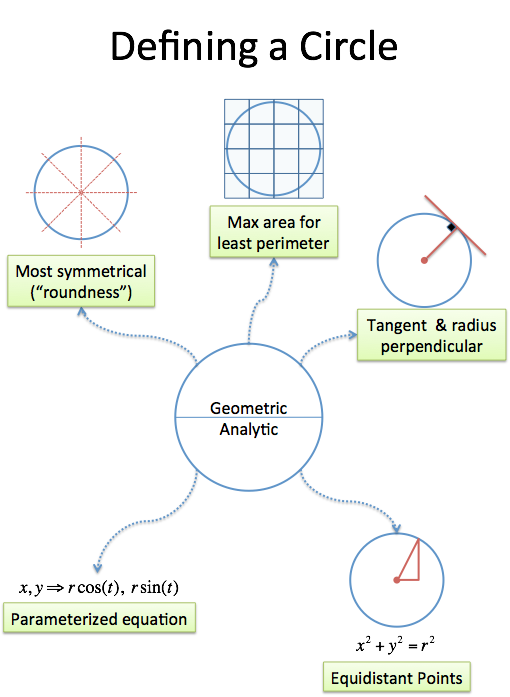There are seemingly countless definitions. Here’s a few:

• The most symmetric 2-d shape possible
• The shape that gets the most area for the least perimeter (see the isoperimeter property)
• All points in a plane the same distance from a given point (drawn with a compass, or a pencil on a string)
• The points (x,y) in the equation x2 + y2 = r2 (analytic version of the geometric definition above)
• The points in the equation r * cos(t), r * sin(t), for all t (really analytic version)
• The shape whose tangent line is always perpendicular to the position vector (physical interpretation)

The list goes on, but here’s the key: the facts all describe the same idea! It’s like saying 1, one, uno, eins, “the solution to 2x + 3 = 5″ or “the number of noses on your face” — just different names for the idea of “unity”.

But these initial descriptions are important — they shape our intuition. Because we see circles in the real world before the classroom, we understand their “roundness”. No matter what fancy equation we see (x2 + y2 = r2), we know deep inside that a circle is “round”. If we graphed that equation and it appeared square, or lopsided, we’d know there was a mistake.

As children, we learn the “caveman” definition of a circle (a really round thing), which gives us a comfortable intuition. We can see that every point on our “round thing” is the same distance from the center. x2 + y2 = r2 is the analytic way of expressing that fact, using the Pythagorean theorem for distance. We started in one corner, with our intuition, and worked our way around to the formal definition.

Other ideas aren’t so lucky. Do we instinctively see the growth of e, or is it an abstract definition? Do we realize the rotation of i, or is it an artificial, useless idea?

## A Strategy For Developing Insight

I still have to remind myself about the deeper meaning of e and i — which seems as absurd as “remembering” that a circle is round or what a cat looks like! It should be the natural insight we start with.

Missing the big picture drives me crazy: math is about ideas — formulas are just a way to express them. Once the central concept is clear, the equations snap into place. Here’s a strategy that has helped me:

• Step 1: Find the central theme of a math concept. This can be difficult, but try starting with its history. Where was the idea first used? What was the discoverer doing? This use may be different from our modern interpretation and application.
• Step 2: Explain a property/fact using the theme. Use the theme to make an analogy to the formal definition. If you’re lucky, you can translate the math equation (x2 + y2 = r2) into a plain-english statement (“All points the same distance from the center”).
• Step 3: Explore related properties using the same theme. Once you have an analogy or interpretation that works, see if it applies to other properties. Sometimes it will, sometimes it won’t (and you’ll need a new insight), but you’d be surprised what you can discover.

Let’s try it out.

## A Real Example: Understanding e

Understanding the number e has been a major battle. e appears all of science, and has numerous definitions, yet rarely clicks in a natural way. Let’s build some insight around this idea. The following section will have several equations, which are simply ways to describe ideas. Even if the equation is gibberish, there’s a plain-english idea behind it.

Here’s a few popular definitions of e: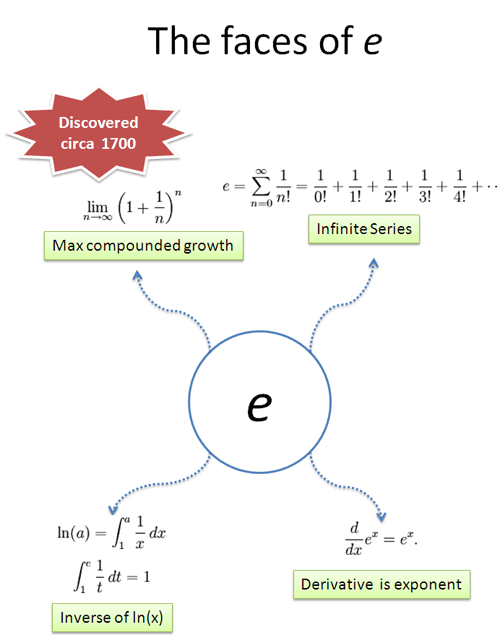The first step is to find a theme. Looking at e’s history, it seems it has something to do with growth or interest rates. e was discovered when performing business calculations (not abstract mathematical conjectures) so “interest” (growth) is a possible theme.

Let’s look at the first definition, in the upper left. The key jump, for me, was to realize how much this looked like the formula for compound interest. In fact, it is the interest formula when you compound 100% interest for 1 unit of time, compounding as fast as possible.

• Definition 1: Define e as 100% compound growth at the smallest increment possible.

The article on e describes this interpretation.

Let’s look at the second definition: an infinite series of terms, getting smaller and smaller. What could this be?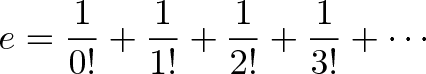$\displaystyle{e = {1 \over 0!} + {1 \over 1!} + {1 \over 2!} + {1 \over 3!} + \cdots}$

After noodling this over using the theme of “interest” we see this definitions shows the components of compound interest. Now, insights don’t come instantly — this insight might strike after brainstorming “What could 1 + 1 + 1/2 + 1/6 + …” represent when talking about growth?”

Well, the first term (1 = 1/0!, remembering that 0! is 1) is your principal, the original amount. The next term (1 = 1/1!) is the “direct” interest you earned — 100% of 1. The next term (0.5 = 1/2!) is the amount of money your interest made (“2nd level interest”). The following term (.1666 = 1/3!) is your “3rd-level interest” — how much money your interest’s interest earned!

Money earns money, which earns money, which earns money, and so on — the sequence separates out these contributions (read the article on e to see how Mr. Blue, Mr. Green & Mr. Red grow independently). There’s much more to say, but that’s the “growth-focused” understanding of that idea.

• Definition 2: Define e by the contributions each piece of interest makes

Neato.

Now to the 3rd, and shortest definition. What does it mean? Instead of thinking “derivative” (which turns your brain into equation-crunching mode), think about what it means. The feeling of the equation. Make it your friend.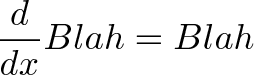$\displaystyle{\frac{d}{dx}Blah = Blah}$

It’s the calculus way of saying “Your rate of growth is equal to your current amount”. Well, growing at your current amount would be a 100% interest rate, right? And by always growing it means you are always calculating interest — it’s another way of describing continuously compound interest!

• Definition 3: Define e as a function that always grows at 100% of your current value

Nice — e is the number where you’re always growing by exactly your current amount (100%), not 1% or 200%.

Time for the last definition — it’s a tricky one. Here’s my interpretation: Instead of describing how much you grew, why not say how long it took?

If you’re at 1 and growing at 100%, it takes 1 unit of time to get from 1 to 2. But once you’re at 2, and growing 100%, it means you’re growing at 2 units per unit time! So it only takes 1/2 unit of time to go from 2 to 3. Going from 3 to 4 only takes 1/3 unit of time, and so on.

The time needed to grow from 1 to A is the time from 1 to 2, 2 to 3, 3 to 4… and so on, until you get to A. The first definition defines the natural log (ln) as shorthand for this “time to grow” computation.

ln(a) is simply the time to grow from 1 to a. We then say that “e” is the number that takes exactly 1 unit of time to grow to. Said another way, e is is the amount of growth after waiting exactly 1 unit of time!

• Definition 4: Define the time needed to grow continuously from 1 to a as ln(a). e is the amount of growth you have after 1 unit of time.

Whablamo! These are four different ways to describe the mysterious e. Once we have the core idea (“e is about 100% continuous growth”), the crazy equations snap into place — it’s possible to translate calculus into English. Math is about ideas!

## What’s the Moral?

In math class, we often start with the last, most complex idea. It’s no wonder we’re confused — we’re showing DNA and expecting students to see the cat.

I’ve learned a few lessons from this approach, and it underlies how I understand and explain math:

• Search for insights and apply them. That first intuitive insight can help everything else snap into place. Start with a definition that makes sense and “walk around the circle” to find others.
• Develop mental toughness. Banging your head against an idea is no fun. If it doesn’t click, come at it from different angles. There’s another book, another article, another person who explains it in a way that makes sense to you.
• It’s ok to be visual. We think of math as rigid and analytic — but visual interpretations are ok! Do what develops your understanding. Imaginary numbers were puzzling until their geometric interpretation came to light, decades after their initial discovery. Looking at equations all day didn’t help mathematicians “get” what they were about.

Math becomes difficult when we emphasize definitions over understanding. Remember that the modern definition is the most advanced step of thought, not necessarily the starting point. Don’t be afraid to approach a concept from a funny angle — figure out the plain-English sentence behind the equation. Happy math.